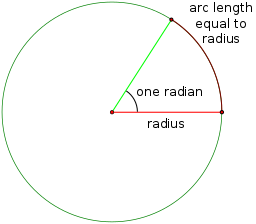# Math Definitions - Letter R

### ChaptersA radian is the angle at the centre of the circle subtended (made) by an arc with the same length as the radius.

There are $2\pi$ radians in a full rotation (once around the circle).

One radian is equal to $\dfrac{180}{\pi}$ degrees, which is approximately equal to $57.3^\circ$.

### Description

The aim of this dictionary is to provide definitions to common mathematical terms. Students learn a new math skill every week at school, sometimes just before they start a new skill, if they want to look at what a specific term means, this is where this dictionary will become handy and a go-to guide for a student.

### Audience

Year 1 to Year 12 students

### Learning Objectives

Learn common math terms starting with letter R

Author: Subject Coach
You must be logged in as Student to ask a Question.